﻿ 水下航行体迎流喷水/气数值模拟研究

水下航行体迎流喷水/气数值模拟研究Numerical Simulation of Jetting Water/Air in the Bow of Underwater Vehicle against the Flow

Abstract: According to Sedov’s theory, this paper put forward the idea of jetting water/air in the bow of un-derwater vehicle against the flow, thus looking forward to reducing the high pressure in the bow and creating air layer to reduce friction resistance at the same time. The flow field around subma-rine is calculated by using Fluent. It shows that, on one hand, the stationary point can be pushed off the submarine by means of jetting water/air against the flow, and get thrust; on the other hand, the surface of submarine can be surrounded by air layer, thus reducing friction resistance. The re-sistance of the submarine can be reduced to 10% - 30% when jetting air/water in the bow of sub-marine against the flow.

1. 引言

2. 数值计算模型的建立

2.1. 模型尺寸及网格划分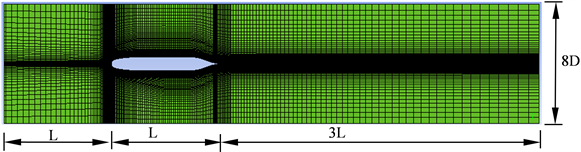Figure 1. The sketch map computational domain and cells

2.2. 边界条件及数值计算方法

2.3. 减阻率与节能率的计算方法

${R}_{t0}={R}_{P0}+{R}_{f0}$ (1)

${R}_{tPj}={R}_{P}+{R}_{i}+{R}_{C}$ (2)

${R}_{C}={\rho }_{j}{v}_{j}^{2}{S}_{j}$ (3)

${R}_{tj}={R}_{tPj}+{R}_{fj}$ (4)

$\mu =\left({R}_{t0}-{R}_{tj}\right)/{R}_{t0}$ (5)

${P}_{S0}={R}_{t0}V$ (6)

${P}_{Sj}={R}_{tj}V+E$ (7)

$E=\left(\frac{{R}_{i}}{{\rho }_{j}}+\frac{1}{2}{v}_{j}^{2}\right){\rho }_{j}Q$ (8)

$\eta =\left({P}_{S0}-{P}_{Sj}\right)/{P}_{S0}$ (9)

3. 水下航行体迎流单相喷水数值模拟

3.1. 喷水口设置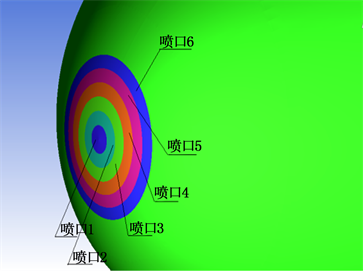Figure 2. The sketch map of submarine at bow

3.2. 水下航行体迎流单相喷水计算结果分析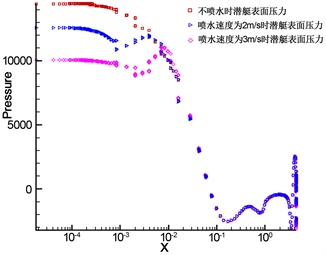Figure 3. Pressure with longitudinal direction x of submarine at different injection velocity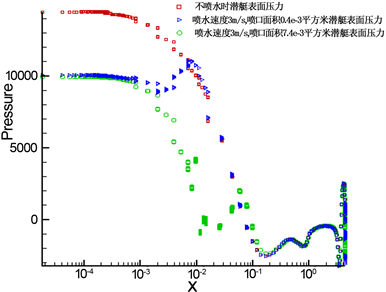Figure 4. Pressure with longitudinal direction x of submarine at different spouts areaTable 2. The result of simulation at different water injection velocityTable 3. The result of simulation at different spouts area (vw = 3 m/s)

4. 水下航行体迎流气液混喷数值模拟

4.1. 水下航行体迎流气液混喷压力分布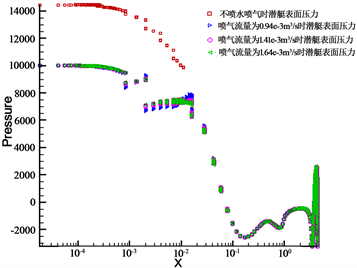Figure 5. Pressure with longitudinal direction x of submarine at different air flow rate (SA3 = 8.10 × 10−3 m2)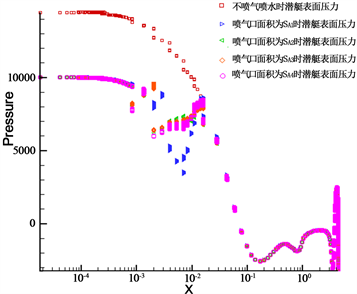Figure 6. Pressure with longitudinal direction x of submarine at different spouts area (Q = 5.83 × 10−3 m3/s)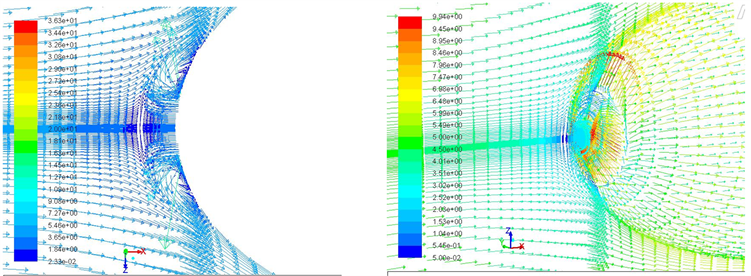Figure 7. Velocity of submarine at different area of jet exhaust (Q = 5.83 × 10−3 m3/s)

4.2. 水下航行体迎流气液混喷阻力计算结果与分析Table 4. The result of simulation at different air flow rate (SA4 = 12.26 × 10−3 m2)Table 5. The result of simulation at different the area of air injection (Q = 13.24 × 10−3 m−3/s)

5. 结论

1) 迎流喷射流体可以使得潜艇艏部驻点脱离潜艇表面前移，从而减小其压差阻力。迎流气液混合喷既可使流动驻点脱离前缘从而降低压差阻力，又可在潜艇表面形成空气润滑，达到减少摩擦阻力的目的。

2) 单相喷水且喷水面积固定时，减阻率以及节能率随喷水速度的增加呈先增后减的变化特点。在喷水速度为3 m/s时减阻率与节能率有最大值，分别为2.61%与2.19%。喷水速度固定时，单相喷水潜艇阻力随喷水面积的增加而减小，减阻率随着喷水面积的增加显著增加，在喷口面积为13.83 × 10−3 m2时减阻率可达60.62%。但减阻效能比随着喷水面积的增加，呈现递减的变化特点。

3) 气液同喷且喷气口面积不变时，摩擦阻力随着喷气流量的增加显著降低。相比仅单相喷水情况，摩擦阻力最大可降低约24%，总阻力最大可降低约20%；喷气流量不变时，摩擦阻力最大可降低约11%，总阻力最大可降低约12%。整体而言，迎流气液同喷的情况下，减阻率与节能率可达到10%~30%。

 盛振邦, 刘应中. 船舶原理[M]. 上海: 上海交通大学出版社, 2008.

 王英奎, 江春波, 李玲. 流动减阻的研究综述[J]. 水力发电, 2008, 34(2): 67-70.

 余永生, 魏庆鼎. 疏水性材料减阻特性实验研究[J]. 实验流体力学, 2005, 19(2): 60-66.

 Gray, J. (1936) Studies in Animal Locomotion: The Propulsive Power of the Dolphin. Experimental Biology, 13, 192-199.

 Toms, B.A. (1948) Some Observations on the Flow of Linear Polymer Solu-tions through Straight Tubes at Large Reynolds Numbers. Proceedings of the First International Congress on Theology, 2, 135-141.

 陈东浩. Toms现象与减阻技术的历史及现状[J]. 化工进展, 1986(1): 32-34.

 л.и.谢多夫. 连续介质力学[M]. 北京: 高等教育出版社, 2004.

 Groves, N.C., Huang, T.T. and Chang, M.S. (1989) Geometric Characteristics of DARPA SUBOFF Models. David Taylor Research Center, Bethesda, Montgomery County, Maryland.

Top